## Sunday, December 25, 2016

### Biggest Science of 2016 - No Evidence of Dark Matter and Dark Energy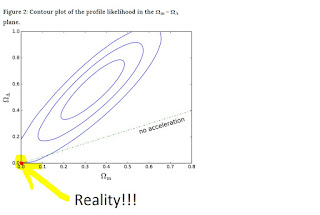Zero Point (0,0) NO DARK MATTER, NO DARK ENERGY:$\Omega_\Lambda$ vs. $\Omega_m$ Universal Reality is pointed out here: No Dark Matter, No Dark Energy
From: nature.com Marginal evidence for cosmic acceleration from Type Ia supernovae

## No Evidence of Dark Matter and Dark Energy

While there is little to no evidence to support dark matter and dark energy, legacy scientists and legacy science still go after these topics with great vigor, investment, publications, and all the fanfare that goes along with the concensous legacy scientific method.

The image above highlights the area with a red dot where our universe lies.  Legacy science claims it's somewhere in the center of the ovals.  This video goes over legacy science's stance:
@ 9:30 The Red Zero Point IS Hyperbolic Black Hole Dynamic Driven SpaceTime!

Legacy science is useful for understanding the fundementals, however, it takes different thinking to solve the unsolved problems than what created them.

The Haramein-Rauscher soultion to Einstein's field equations combined with their other work at https://hiup.org/publications and course work at Resonance Academy and all the many hours of lectures/videos over many decades revisit the fundamentals of the two main science theories
1. Cosmology: Einstein (et al), General Relativity (GR)
2. Quantum: Planck (et al), The Standard Model (SM)

and gives great insight into how Nature truly behaves (superfluid like aether, tetrahedral structure, holographic, connected, alive/conscious - blueprint for life IS in the vacuum).

Perhaps more time should be invested in comparing the results of legacy analysis of Dark Matter and Dark Energy plots $\Omega_\Lambda$ vs. $\Omega_m$, comparing to GR using Torque and Coriolis, to determine a similar plot to $\Omega_\Lambda$ vs. $\Omega_m$ however, using Torque and Coriolis.  Considering that the legacy science isn't whole, often it is better to invest the time in the ideas that work rather than working with a failed theory - however, it can be instructive.  Anyway, we move on, as this blog is to point out viable scientific and consciousness ideas that address science and the long unsolved physics problems (I consider physics to be all encompassing, including consciusness as well.  Complete physics includes ALL).

While the Dark Matter and Dark Energy failure is ongoing, not enough effort/publicity is placed on the geometric nature of the structure of the Universe:red square nebula MWC 922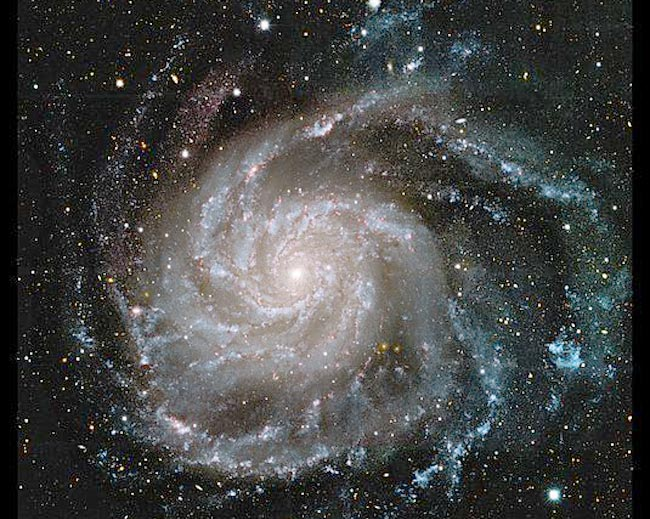Pinwheel galaxy M101 has square arms like our milky wayHexagonal galaxy http://www.caelumobservatory.com/obs/m61.html
More galaxy images here:  (many have geometric shape, about > 80%)Sacred geometry example (phi-spiral implosion / resonance - cymatics)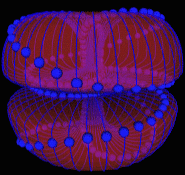Dual-tours black hole dynamics driven aether - simplified.  Actual pattern more complex.Someone's been attempting to tell us...

Anyway, what I'm saying is there is much evidence to support a geometric universe, overwhelming evidence.  There are galaxies of all types of geometric shapes, and it is fits well with the ancient sacred geometry teachings.

My best guess on how our Universe behaves is a combination of Haramein-Rauscher with The Electric Universe (Maxwell or EM theory added to GR, which it already is if one decides to focus on those terms) and Platonic nested phi-ratioed sacred geometries - it's holofractal, Hermetic, as above, so below. A superfluid aether is needed to be added back to advance the physics.

What I would look out for in 2017 and the future is the resolution of the proton radius problem:
2. more later...
and how as this problem is resolved that insight is gained into the vacuum (vacuum catastrophe) which will lead right to the fundamental structure of the Universe and resolve Dark Matter and Energy and explain Galaxtic structure and star speed profiles and much more (gravitational control and energy/matter from/into the vacuum).

We are on the cusp of great revelations.

The Surfer, OM-IV

# It's official: NASA's peer-reviewed EM Drive paper has finally been published

Measurement of Impulsive Thrust from a Closed Radio-Frequency Cavity in Vacuum Read More: http://arc.aiaa.org/doi/10.2514/1.B36120

After reading their hypothesis for how the EM Drive could produce thrust, it is clear the "official NASA science" explanation IS EXACTLY WHAT Nassim Haramein has been saying for about his whole lifetime:
If the vacuum is indeed mutable and degradable as was explored, then it might be possible to do/extract work on/from the vacuum, and thereby be possible to push off of the quantum vacuum and preserve the laws of conservation of energy and conservation of momentum. It is proposed that the tapered RF test article pushes off of quantum vacuum fluctuations, and the thruster generates a volumetric body force and moves in one direction while a wake is established in the quantum vacuum that moves in the other direction.

see 10. Discussion  for more detail of the explanation. Uses pilot wave theory and Bohm's arguements.  Basically, adding back the aether to physics and saying one can push of the vacuum, just like I said in my earlier post:

## Thursday, April 30, 2015 -

So, once the underlying physics is better understood, gradients in the vacuum can be optimsed for greater thrust and energy extraction.  It just a matter of time before the mainstream catches up and helps to develop the theory and bridge it into engineering from that theory.  Much work has already been done by the alternative community.

More later.  New things are coming.  Great things. A Connected Universe.

The Surfer, OM-IV

## Saturday, September 24, 2016

### The Connected Universe

We are proud to announce that Sir Patrick Stewart, known for his role as Star Trek: The Next Generation's Captain Jean-Luc Picard, is the narrator of The Connected Universe, a groundbreaking feature film documentary by Malcom Carter. Watch the newly updated trailer featuring the voice of Patrick Stewart and get tickets to the premier in L.A. on Monday 9/26/2016 –> http://getconnected.resonance.is "The Connected Universe” explores the interconnection of all things in the Universe. It inspires people to see the world differently. There is no better guide on this journey of exploration than Sir Patrick Stewart. Known for boldly exploring the galaxy in Star Trek, he is also revered as a Royal Shakespearean actor who has taken an inner journey of extraordinary depth. This intertwining of both science and humanity creates a unique narrative to explore connection. Nassim Haramein • Resonance Science Foundation - Français • Resonance Science Foundation - Hispana • Resonance Science Foundation - Português • Collective Evolution • Unify • Thrive • Spirit Science • Universe Explorers • Physics-Astronomy • Resonance Science Foundation - 中文 • Cosmometry

## Thursday, September 8, 2016

### Light and Smoke Rings

Spatiotemporal optical vortices, or STOVs (thin, gray ringlike objects), are newly described three-dimensional light structures that strongly resemble smoke rings.

Interesting.

Remember my past post about smoke rings:

Different, however, related concepts.

Considering that the aether is a superfluid of immense density, something moving through it will leave vortices.  The concept is important, and this photonic vortex may be supporting this concept.
And light or photons, being electro-magnetic radiation is itself an othogoanl votrex of E and M radiation, which is a relativistic projection of a single field, just looks like two fields due to motion (Feynman mentions this in his reds rags, buried in there somewhere: http://www.feynmanlectures.caltech.edu/II_01.html).

As above, so below:

Nature behaves in ways not yet captured by mainstream science.  They're getting closer...

The Surfer, OM-IV

## Tuesday, August 16, 2016

### Tinkering and Hammering Out Equations

This is how it's done.

So, you're wondering, "hey, exactly how are you going to crank out these equations people have been looking for for likely hundreds of years over the next few months?"  Well, just like I said way back in the beginning of the blog, we'll do it the old fashioned way, and that is step by step.

Alternative method:

The Surfer, OM-IV

## Monday, August 15, 2016

### We're Calculating Masses of Fundamental Particles Now!!!

We can now calculate, from first principles and fundamental Planck units, the mass of blackholes, protons, and electrons. (link to post about new electron holographic solution)

The proton actually is a fundamental particle as well.  The Standard Model considers that the proton is composed of quarks, thus not fundamental.

So, now, what about all of the other fundamental particles?  Can we also calculate their masses and predict all their masses correctly, and predict masses of other unknown particles?

For all the success of The Standard Model, it is incomplete, and, while it works very well for some things (QED, QCD, etc), it has issues.

Anyway, we can now calculate the mass of one of the elementary particles in the table above, the electron.  It seems then like the muon or tau particle would be the appropriate particle to target next to see if we can calculate its mass, and perhaps along the way determine or demonstrate exactly what works and what does not work with "The Standard Model" way of looking at things.

For now, we will list the masses of the first three leptons:
 electron mass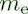Value 9.109 383 56 x 10-31 kgStandard uncertainty 0.000 000 11 x 10-31 kgRelative standard uncertainty 1.2 x 10-8Concise form 9.109 383 56(11) x 10-31 kg

 muon mass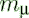Value 1.883 531 594 x 10-28 kgStandard uncertainty 0.000 000 048 x 10-28 kgRelative standard uncertainty 2.5 x 10-8Concise form 1.883 531 594(48) x 10-28 kg

 tau mass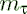Value 3.167 47 x 10-27 kgStandard uncertainty 0.000 29 x 10-27 kgRelative standard uncertainty 9.0 x 10-5Concise form 3.167 47(29) x 10-27 kg

And their relative mass ratios:

 muon-electron mass ratio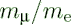Value 206.768 2826

 tau-electron mass ratio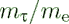Value 3477.15

 tau-muon mass ratio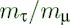Value 16.8167

Since the muon and tau are simply more energetic electrons, it seems like if these were actual particles, we should be able to find an equation that predicts their masses based upon first principles and fundamental Planck constants.

To do this, we will have to look more deeply into the muon and tau particles.  We are having great success so far at applying Haramein's Holographic Solution, and the approach will either solve for the masses of these other particles, or explain what is wrong with The Standard Model.

Since this work is too large to fit in the margin of this post, we'll do it over the next few posts, and months, until some resolution is made.

The writing is already on the wall that The Standard Model has missed the boat, while at the same time MISSING a whole lot of things that really matter.

That's all for now.

The Surfer, OM-IV

## Sunday, August 14, 2016

### Another Equation for Proton to Electron Mass Ratio???

$$\mu={m_p\over m_e}={2\phi m_{\ell}\over {\phi_em_{\ell}/2\alpha}}=4\alpha{\phi\over\phi_e}=4\alpha{a_0\over r_p}=1836.15267...$$
Compare to:
$$\mu={\alpha^2\over{\pi r_pR_{\infty}}}=1836.15267...$$
$\mu=$ proton to electron  mass ratio
$m_p=$ mass of proton
$m_e=$ mass of electron
$\phi={\eta\over R}$ Holographic ratio for proton
$\phi_e={\eta_e\over R_e}$ Holographic ratio for electron
$m_{\ell}=$ Planck mass
$a_0=$ Bohr radius
$r_p=$ proton radius (muonic hydrogen proton radius)
$R_{\infty}=$ Rydberg constant

((4 * fine-structure constant * hbar) / (m_e * c * fine-structure constant)) / (((4 * hbar) / c) / m_p) =
1 836.15267

CODATA value for proton-electron mass ratio:
http://physics.nist.gov/cgi-bin/cuu/Value?mpsme
 proton-electron mass ratio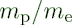Value 1836.152 673 89Standard uncertainty 0.000 000 17Relative standard uncertainty 9.5 x 10-11Concise form 1836.152 673 89(17)

There remain a few issues to be ironed out as Haramein's paper, The Electron and the Holographic Mass Solution, reports the proton to electron mass ratio as:
$$\mu={m_p\over m_e}={2\phi m_{\ell}\over {\phi_em_{\ell}/2\alpha}}=4\alpha{\phi\over\phi_e}=1836.942579077855...$$

So, the work continues to understand this difference...

This result, the 1836.94259077855... above, is calculated using the CODATA value for $\alpha$, the 2013 muonic hydrogen charge radius in the $phi$ calculation, and $phi_e=6.108458512E-25$ .  See Google Drive Excel File for calculations.

More in upcoming post: Another Equation for Proton to Electron Mass Ratio!!! #2

The Surfer, OM-IV

## Saturday, August 13, 2016

### The Electron and the Holographic Mass Solution #2

Sword of Science - SoS

...continuing with Haramein's The Electron and the Holographic Mass Solution:
Let's examine the expression for the electron mass and go through the exercise of how to put numbers to the equations and compare the results to CODATA measurements and previous mainstream theoretical predictions and analysis.
$$m_e={1 \over 2\alpha}\phi_e m_\ell$$
$m_e=$ mass of the electron
$m_\ell=$ Planck mass
$\phi_e={\eta_e\over R_e}=$ electron Holographic ratio
$\eta_e=$ Surface entropy
$R_e=$ Volume entropy
$\alpha=$ fine-structure constant

The subscript "e", for electron, is to emphasize that the $\eta_e$ and $R_e$ are to be calculated for the electron, using the confined electron's Bohr hydrogen radius atomic volume and the surface area to calculate $\eta$ and $R$.

Let's do $\eta$, the surface entropy first:
$$\eta=\eta_e$$
$$\eta_e={A_{e-surface}\over A_{eq}}$$
$$A_{e-surface}=4\pi {a_{0}}^2$$
$$A_{eq-PSU}=\pi {r_{\ell}}^2$$
$a_0=$ Bohr hydrogen radius - distance electron is away from proton center
$r_{\ell}$ is the Planck radius of the PSU
$r_{\ell}={\ell\over 2}$
$\ell=$ Planck length
$A_{e-surface}$ is the surface area of a sphere of radius $a_0$, the Bohr radius.
$A_{eq-PSU}$ is the equatorial cross-sectional area of the Planck Spherical Unit (PSU).

$$\eta=\eta_e={4\pi {a_{0}}^2\over \pi {r_{\ell}}^2}$$

$R_e$, the volume entropy is next:
$$R_e={V_e\over V_{PSU}}$$
$$R_e={{4\over3}\pi a_0^3\over {4\over3}\pi r_{\ell}^3}={a_0^3\over r_{\ell}^3}$$

And finally:
$$\phi_e={\eta_e\over R_e}$$
$$\phi_e={4r_{\ell}\over a_0}$$
$$m_e={{1\over2\alpha}\phi_em_{\ell}}$$

electron mass =
9.10938356 × 10-31 kilograms

CODATA Link to M_e electron mass
 electron massValue 9.109 383 56 x 10-31 kgStandard uncertainty 0.000 000 11 x 10-31 kgRelative standard uncertainty 1.2 x 10-8Concise form 9.109 383 56(11) x 10-31 kg

Check!!!  Another success of Haramein's holographic approach.

Some further simplifications/reductions:
$$m_e={{1\over2\alpha}{4r_{\ell}\over a_0}m_{\ell}}$$
$$m_e={\ell m_{\ell}\over\alpha a_0}$$
$$\ell=\sqrt{\hbar G \over c^3}$$
$$m_{\ell}=\sqrt{\hbar c\over G}$$
$${\ell}m_{\ell}={\hbar\over c}$$

$$m_e={\hbar\over c\alpha a_0}$$
$$a_0={\hbar\over m_ec\alpha}$$

The Surfer, OM-IV

## Friday, August 12, 2016

### The Revolution in Particle Physics continues!!!

It will be interesting to see how it all unfolds.  A few recent online articles mention a third verification of the proton radius problem, physics definately is in trouble:

Two areas will be opened by the unified physics:
1. Gravitational control (anti-grav)
2. ZPE, or energy extraction from the vacuum (and matter creation as well)
And look at all of the long unsolved physics problems and mysteries and paradoxes that are slowly, step by step, being explained by the unified physics of Haramein.

The Revolution in Particle Physics continues!!!
The Surfer, OM-IV

### Nassim Haramein: The Electron and the Holographic Mass Solution #1

Nassim Haramein and Amira K. F. Val Baker have written a paper that extends Haramein's holographic mass technique/approach to the electron.  This approach is derived from first principles and fundamental Planck units based on a geometric and informetric/entropic ratios.

From http://hiup.org/publications/: (link to Haramein's publication)

#### The Electron and the Holographic Mass Solution

Haramein, N & Val Baker, A. K. F. (2016). The Electron and the Holographic Mass Solution, ATINER 4th International Conference of Physics: Abstract Book, Page 14

Let's look at the black hole, proton, and the new electron mass equations, in general form before we break it down into specifics:
$$m_{BH}={m_\ell \over \phi}$$
$$m_p={2\phi m_\ell}$$
$$m_e={1 \over 2\alpha}\phi_e m_\ell$$
$m_{BH}=$ Schwarzschild mass of the black hole
$m_p=$ mass of the proton
$m_e=$ mass of the electron
$m_\ell=$ Planck mass
$\phi={\eta\over R}=$ Holographic ratio
$\phi_e={\eta\over R}=$ electron Holographic ratio
$\eta=$ Surface entropy
$R=$ Volume entropy
$\alpha=$ fine-structure constant
The general form for mass is:
$$mass=f(\phi)m_\ell$$
where we simply need to know the function of $\phi$ required for the object in question. Determining this function phi requires a little insight into the nature of the object and the geometric and informetric ratios.  This is an area we'll explore more later.
The solution for the electron proceeds exactly the same as for the black hole and the proton, basically calculating $\eta$ and $R$ using the Bohr radius, $a_0$, for the electron.  Details are in the paper linked above, and we will go over it more in later posts.
Significantly, in addition to the electron mass, Haramein has provided new, more accurate DERIVATIONS of the Rydberg constant, fine-structure constant, and proton to electron mass ratio equation and the derivation and his approach gives insight into the origin and nature of the constants and may even be able to address "discrepancies between experimental and theoretical values."
This cannot be emphasized enough how significant it is, the insight Haramein's analysis gives into these constants and the source and nature of mass of matter.
Over the next few posts we'll go into more detail, as this is enough for now.

The Revolution in Particle Physics continues!!!
The Surfer, OM-IV

## Saturday, August 6, 2016

### COLD FUSION DISCOVERY

FYI:  #ColdFusion #LENR is for real.  This "new" effect has been suppressed by the "mainstream".

The mainstream scientist can't explain how it works because it requires the new physics to understand how it works.  (Nassim Haramein's theory combined with Dan Winter's optimization).

This technology is useful, however, there other other ZPE devices possible in the framework of the new physics that the mainstream is ignoring.

(the new physics is almost the same as the old mainstream physics, it simply requires a few new basic "assumptions" that connect everything - the big and the small)

It is a #transmutation process #Alchemy is for real. #Newton.

# SPECIAL REPORT: GOV'T HIDING COLD FUSION DISCOVERY

For a mainstream scientist to quickly see how the "threshold" of the reaction can be attained, consider phonons, phononic vibrations shaking the matrix, and the density of the matrix is the aether, with a density of the Planck mass to the Planck Spherical Units volume (PSU) which is VERY HIGH DENSITY of ENERGY per volume, so a little shaking goes along way interacting with the catalytic like doping layers that assist the Hydrogen transmutation to He, as well as other transmutations.

It's ideas like this that power James Gilliland's ships.

The Surfer, OM-IV

## Friday, July 29, 2016

### Additional Notes on Full Derivation of Proton to Electron Mass Ratio

The derivation used a combination of this approach I've outlined by inspecting Maxwell's and Schrodinger's equations AND as I mentioned in later posts, a mathematical approach:

http://phxmarker.blogspot.com/2016/03/derivation-of-proton-to-electron-mass.html

All that combined, then one can reproduce all the steps. And if I recall correctly, the equations really behind this are Maxwell's equations with a modification I made to examine both proton and electron, thus, in my opinion, the Electric Universe Theory is key to be included with the new unified physics.﻿

Thunderbolts of the Gods | Official Movie

Oh, yes, and VERY BIG NEWS!!! To be reported on by the end of this year.  Nassim Haramein has applied his technique to the electron AND GETS VERY ACCURATE ANSWERS that agree with electron mass measurements.  He also mentioned the eso-teric proton to electron mass ratio equation, consciouness, and a lot more.  His papers and analysis will be made available later this year according to the Live with Nassim talk at about 1 hour into the video (Resonace Academy members only - link at side of blog for those interested and I get a percentage to help support this blog).

The Surfer, OM-IV

## Wednesday, July 6, 2016

### The Connected Universe | Nassim Haramein | TEDxUCSD

The Connected Universe | Nassim Haramein | TEDxUCSD

"It's a whole new world!" -Simon, when a new version of Minecraft comes out.  Likewise, when Haramein's physics gets out.

The Surfer, OM-IV